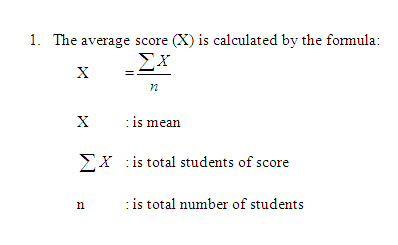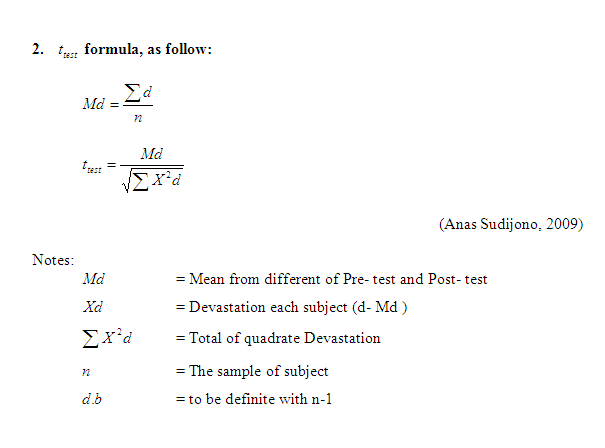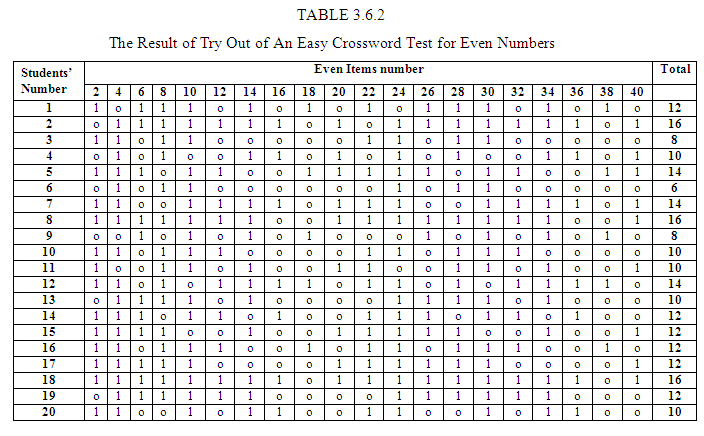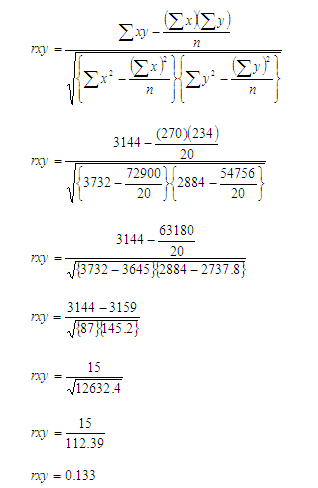# Contoh Skripsi Bahasa Inggris Chapter III Tentang Crossword Dan Vocabulary

Posted on

## Contoh Skripsi Bahasa Inggris Chapter III Tentang Crossword Dan Vocabulary### CHAPTER III THE METHOD OF THE RESEARCH

#### 3.1 Method of the Research

The research was conducted by using the experimental method to know the influence of An Easy Crossword toward students’ vocabulary mastery, the writer used a class. The writer did the treatment to know the influence of an Easy Crossword towards students’ vocabulary mastery. The treatment was done four times. After giving the treatment the writer gave the test.

#### 3.2 The Variable of the Research

In this research there are two variables, as follow:

1. The independent variable is An Easy Crossword (X).
2. The dependent variable is students’ vocabulary mastery (Y).

#### 3.3 The Operational Definition of Variable

The operational definition of the variable is used to describe the characteristics of the variable that was investigated by the researcher:
1. An Easy Crossword is the teaching technique that designed to improve students’ vocabulary mastery.
2. Students’ vocabulary mastery is that knowing the meaning of English words and being able to use the words appropriately various context or the student’s knowledge about vocabulary which knows the meanings of the words and able to use it. It is indicated by the score achieved from the test given.

#### 3.4. The Population, the Sample, and the Sampling Technique

##### 3.4.1 The Population
In this research, the students of SMP Budi Mulia Kedaton, Bandar Lampung at the second semester in academic year 2009/2010 had a class of class seventh. It consists of 39 students. Total populations were 39 students.##### 3.4.2 The Sample
The writer took only one class because there is only one class in SMP Budi Mulia Kedaton, Bandar Lampung at the second semester in academic year 2009/2010.

##### 3.4.3 The Sampling Technique
Conducting the research, the writer used Total Sampling Technique. It means that based on the population and sample the writer took the sample totality because there is only one class of the seventh class.

#### 3.5 The Data Collecting Technique

In the research, the writer needed some data to complete the research. The writer used some technique for collecting data, that is:

1. The Main Technique

In this research, the writer gave the students a test. The test is multiple choices to know the students’ vocabulary mastery.

2. The Supporting Technique

In order to be able to collect the data, the researcher uses observation to find out appropriate data, the writer uses the observation to know the condition of the school, the material of the students themselves. In this case, the writer saw the way of teaching and learning the process in the class especially in English.

#### 3.6 The Validity and Reliability of Test

##### 3.6. 1 The Instrument Test of Research
###### 3.6.1. 1 The Validity of Test
A test is said as a good test if a test processes the validity. Validity means the validity of the test exactly measure what it is meant to measure. To know the validity of the test, in this research, the researcher used the current curriculum. It means that the test is designed based on the current and curriculum. To determine the reliability of the test, the researcher tried out test items.

###### 3.6.1. 2 The Reliability of Test
To know the reliability of the test, the writer did the tryout. The sample is out of the sample. The steps of doing the try out are as follow:
1. Perform the test to 20 students.
2. Divide the result into even and odd member.
3. Analyze the result by using Product Moment Formula as follow:4.   For determining reliability test, the writer did the try out first, used the formula of Sperman Brown as follows:#### 3.7 The Data Analysis

To know the result of the research, the research analysis the data. In analyzing the data of this research, the researchers used the mean recommended.

This is represented by the following formula:Contoh Skripsi Bahasa Inggris Chapter III Tentang Crossword Dan VocabularyTo find out the correlation between odd and even group, the writer used the product moment formula as follow:Then to determine the reliability of using An Easy Crossword, the writer continued calculating by using Spearman-Brown as follows:Based on the calculation of trying out above, the implementation of using An Easy Crossword is showed that the coefficient correlation is 0.22. It means that the test item of An Easy Crossword can be used in this research.

Simak Chapter selanjutnya di sini:

Semoga artikel ini membantu teman-teman semua dalam pembuatan Skripsi khususnya Pendidikan Bahasa Inggris.

Vote your voice for better articles

Best regards: PustakaBahasaInggris.com